Home Practice
For learners and parents For teachers and schools
Textbooks
Full catalogue
Pricing SupportLog in

We think you are located in United States. Is this correct?

# Vertically opposite angles

## 12.3 Vertically opposite angles

In the diagram below, two straight lines intersect at a point, forming the four angles $$\hat{a}, \hat{b}, \hat{c} , \text {and } \hat{d}$$.

• $$\hat{a}$$ is vertically opposite $$\hat{c}$$, and
• $$\hat{b}$$ is vertically opposite $$\hat{d}$$.
intersect
cross each other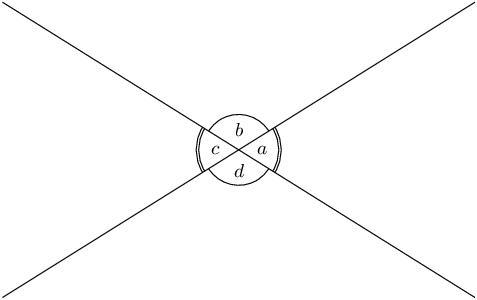Vertically opposite angles (vert opp $$\angle$$s) are the angles opposite each other when two lines intersect.

vertically opposite angles
angles that lie opposite each other when two lines intersect, and are therefore equal in size

Vertically opposite angles are always equal. In the diagram above, $$\hat{a}=\hat{c}$$ and $$\hat{b}=\hat{d}$$.

All the angles around one point form a revolution. One revolution is equal to $$360^{\circ}$$.

In the diagram below, the three angles $$\hat{a}$$, $$\hat{ b}$$, and $$\hat{c}$$ form a revolution.

$$\hat{a}+\hat{b}+\hat{c}= 360^{\circ}$$ ($$\angle$$s around a point)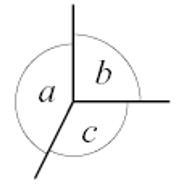## Worked example 12.4: Calculating unknown angles when two lines intersect

Calculate the size of $$x$$, $$y$$ and $$z$$.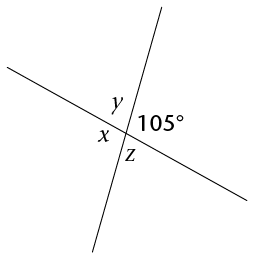### Identify the vertically opposite angles in the diagram.

Here we can see that:

• $$x$$ is vertically opposite $$105^{\circ}$$, so $$x=105^{\circ}$$
• $$y$$ is vertically opposite $$z$$, and therefore $$y=z$$

### Identify the adjacent angles on each straight line in the diagram.

$$y$$ and $$105^{\circ}$$ are angles on the same side of the straight line, so their sum must be $$180^{\circ}$$.

### Use the above information to calculate the sizes of all the angles.

\begin{align} x &= \text{105}^{\circ} &&(\text{vert opp }\angle\text{s}) \\ y + 105^{\circ} &= \text{180}^{\circ} &&(\angle\text{s on a straight line}) \\ y &= \text{180}^{\circ} - 105^{\circ} && \\ & = \text{75}^{\circ} \\ z &= \text{75}^{\circ} &&[\text{vert opp }\angle\text{s}] \end{align}

## Worked example 12.5: Equations using vertically opposite angles

Calculate the size of $$m$$.

### In this diagram, we see that the two marked angles lie vertically opposite each other. So, the two marked angles must be equal.

We can write out an equation, and then solve for $$m$$.

\begin{align} m + 20^{\circ} &= 100^{\circ}&& (\text{vert opp }\angle\text{s}) \\ m &= 100^{\circ} - 20^{\circ} \\ &= 80^{\circ} \end{align}

## Worked example 12.6: Equations using angles around a point

Calculate the size of $$y$$, giving a reason for your answer.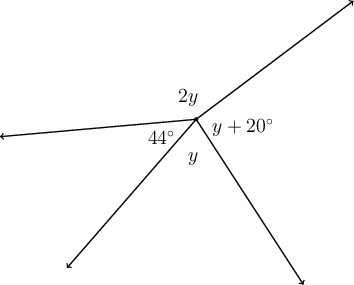### Identify all the angles around the given point.

In this diagram, we see that the four marked angles are all angles around the same point. We will use the fact that angles around a point add up to $$360^{\circ}$$ to form an equation to help us solve for $$y$$.

### Write out an equation, and then solve for $$y$$.

\begin{align} 2y + 44^{\circ}+y + 20^{\circ}+y &= 360^{\circ}&& (\angle \text{‘s around a pt.}) \\ 4y + 64^{\circ} &= 360^{\circ} \\ 4y &= 360^{\circ} - 64^{\circ} \\ 4y &= 296^{\circ} \\ y &= 74^{\circ} \end{align}
Exercise 12.2

Calculate the size of $$j$$, $$k$$ and $$l$$.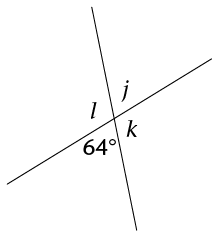\begin{align} j &=64^{\circ} & (\text{vert opp }\angle\text{s}) \\ k+64^{\circ} &=180^{\circ} &(\angle\text{s on a straight line}) \\ k&=180^{\circ} - 64^{\circ} \\ k&=116^{\circ} \\ l &=116^{\circ} & (\text{vert opp }\angle\text{s}) \end{align}

Calculate the size of $$a$$, $$b$$, $$c$$ and $$d$$.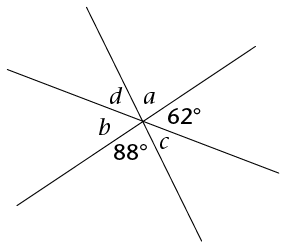\begin{align} a &=88^{\circ} & (\text{vert opp }\angle\text{s}) \\ b &=62^{\circ} & (\text{vert opp }\angle\text{s}) \\ 88^{\circ}+c+62^{\circ} &=180^{\circ} &(\angle\text{s on a straight line}) \\ c+150^{\circ} &=180^{\circ} \\ c &=180^{\circ} - 150^{\circ} \\ c&=30^{\circ} \\ d &=30^{\circ} & (\text{vert opp }\angle\text{s}) \end{align}

Calculate the size of $$t$$.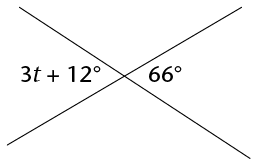\begin{align} 3t + 12^{\circ} &=66^{\circ} & (\text{vert opp }\angle\text{s}) \\ 3t &=66^{\circ} - 12^{\circ} \\ 3t &=54^{\circ} \\ t &=18^{\circ} \end{align}

Calculate the size of $$p$$.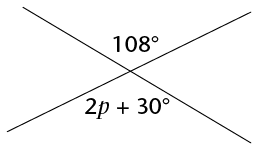\begin{align} 2p+30^{\circ} &=108^{\circ} & (\text{vert opp }\angle\text{s}) \\ 2p &=180^{\circ}-32^{\circ} \\ 2p &=148^{\circ} \\ p &=74^{\circ} \end{align}